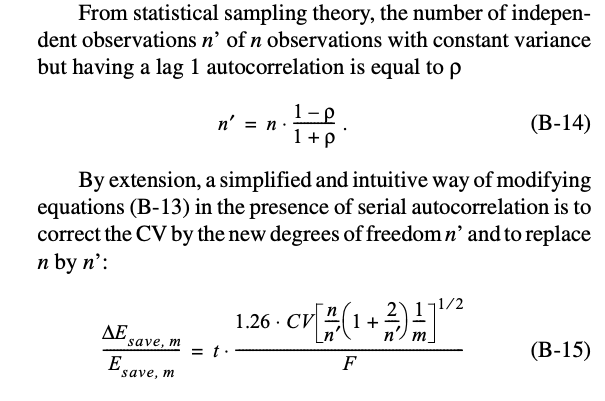Question-and-Answer Resource for the Building Energy Modeling Community
Get started with the Help page

M&V Model Statistical Uncertainty under ASHRAE 14I have a question about how to use ASHRAE 14's formula for calculating energy savings model uncertainty:where n and m are "number of observations in the baseline (or pre- retrofit) and the post-ECM periods, respectively"

So if the model is fitted with daily data, should n be 365? If the model is used to compare energy savings over a year, should m be 365? Or should m be 30 if we're comparing the energy savings on a monthly basis?

What about a model based on hourly data? Should n be 24*365 = 8760? What should m be if we use an hourly model?

edit retag close merge delete

Sort by » oldest newest most voted

I interpret equation B-15 to mean this:

• If you have 365 samples of pre-retrofit data: n = 365. (Example: daily meter data, one year)
• If you have 8760 samples of pre-retrofit data: n = 8760 (Example: hourly meter data, one year)
• If you have 30 samples of post-retrofit data: m = 30 (Example: daily meter data, one month)

Recall that the assumption with this section is that you have applied the pre-retrofit model (based on the n samples) to the actual weather data in the post-retrofit period. I think your question indicates the same interpretation as I have.

Regarding getting to this equation: it seems to come from some of the references like Reddy and Claridge, 2000, Uncertainty of "Measured” Energy Savings from Statistical Baseline Models, appearing in ASHRAE HVAC&R Research. The derivation refers to "numerical trials" so that may come out differently based on the source of the numbers used in said trials. That said, it also reads "m = number of periods (hour, day, or month) in the post-retrofit period," so that supports our interpretation that it is meant to be generally applicable, anyway.

In the wild, I've seen a couple of variations on this formula:

• In place of the magic number 1.26, ECAM uses a quadratic expression, I think.
• Authors of RMV2.0 have a comment on Github issue #7 that explains why they dropped the uncertainty calcs, based on equation B-15, for their TOWT model.

Since there appears to be some dissent, it seems prudent to use this equation with caution. Annex B glosses over lots of details regarding uncertainty, and I think a user's guide would be very helpful.

more